# What is a Fraction?

## Fractions represent equal parts of a whole or a collection.

Fraction of a whole: When we divide a whole into equal parts, each part is a fraction of the whole.

For example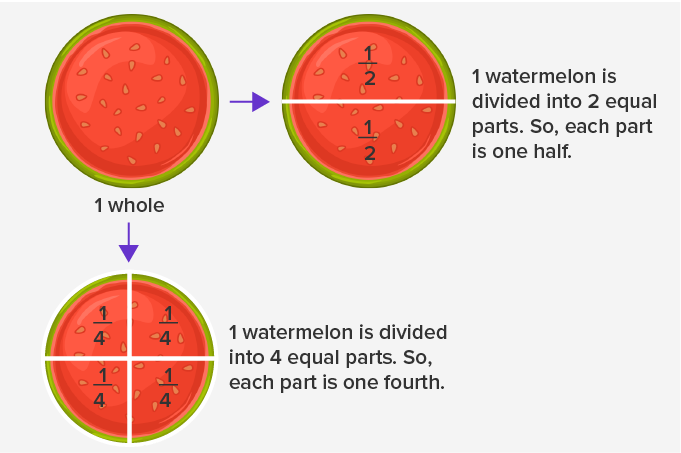Fraction of a collection: Fractions also represent parts of a set or collection.

For example

There is a total of 5 children.

3 out of 5 are girls. So, the fraction of girls is three-fifths ( 35 ).

2 out of 5 are boys. So, the fraction of boys is two-fifths ( 25 ).

## Fraction notation

A fraction has two parts. The number on the top of the line is called the numerator. It tells how many equal parts of the whole or collection are taken.  The number below the line is called the denominator.  It shows the total divisible number of equal parts the whole into or the total number of equal parts which are there in a collection.Fractions on a number line: Fractions can be represented on a number line, as shown below.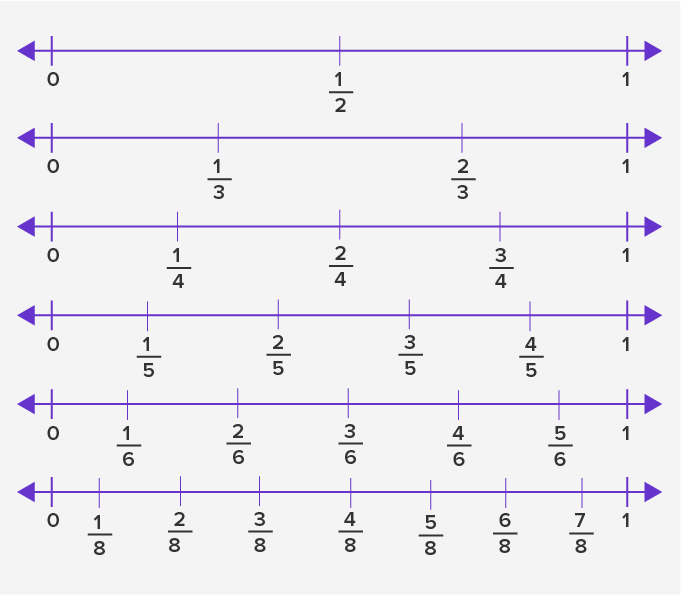For examples,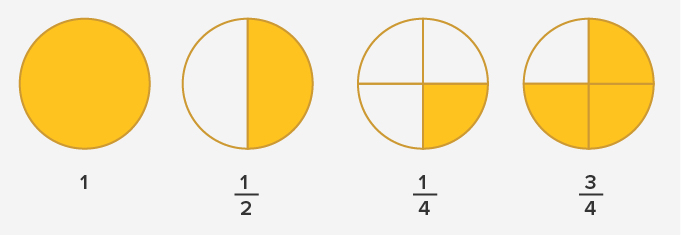## Real-life examples

The most common examples of fractions from real life are equal slices of pizza, fruit, cake, a bar of chocolate, etc.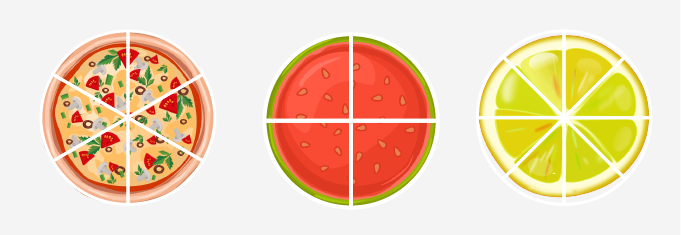## Non-examples

When the parts of the whole are unevenly divided, they don’t form fractions.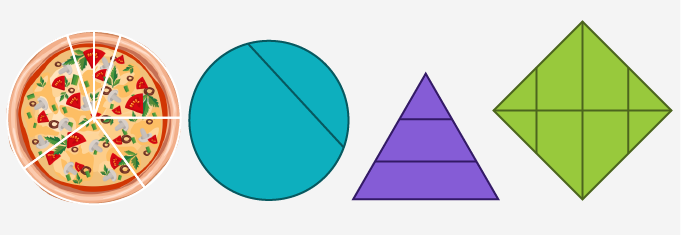## Types of fractions

### Unit fractions

Fractions with numerator 1 are called unit fractions.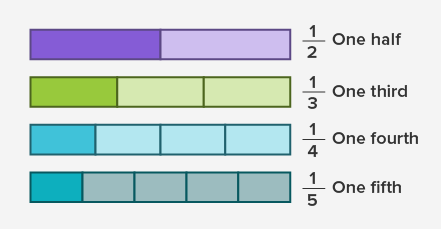### Proper fractions

Fractions in which the numerator is less than the denominator are called proper fractions.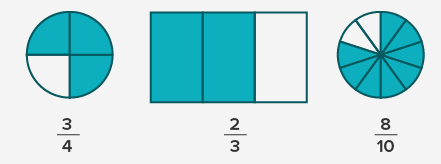### Improper fractions

Fractions in which the numerator is more than or equal to the denominator are called improper fractions.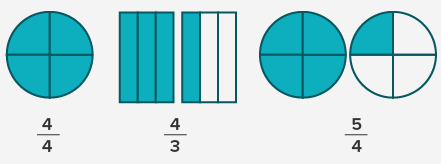### Mixed fractions

Mixed fractions consist of a whole number along with a proper fraction.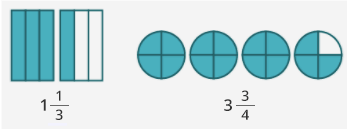## Fun Facts

• The word ‘fraction’ has been derived from the Latin ‘fractus’ which means “broken”.

• Fraction has been into existence from the Egyptian era which is known to be one of the oldest civilizations in the world. However, fractions were not regarded as numbers, in fact, they were used to compare the whole numbers with one another.
Previous Post Next Post